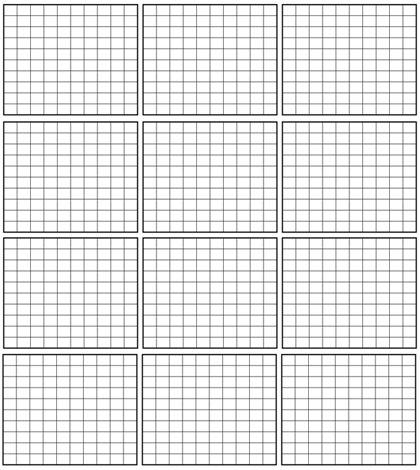## Monday, January 6, 2014

### An Exercise for the Mind: a 10 by 10 Math Puzzle: a Pattern Recognition Game: Meditation on an Open Maze

A few years ago one of my students introduced me to a simple and intriguing game. He didn’t know where the game originated from but said that it was popular in Turkey, and that he and his friends loved playing it.

It is probably best categorised as a mathematical puzzle that requires no arithmetic calculations, just placements of numbers based on two rules; hence I prefer to refer to this puzzle as a pattern recognition game, or simply, an exercise for the mind using an open maze – a meditation of sorts.

The Purpose of the Game
To take a 10 by 10 grid, equaling 100 squares, and completely filling in every square based on two types of movements.

The Rules are Simple
1) Create a 10 by 10 grid (graph paper or a spread sheet will come in very handy).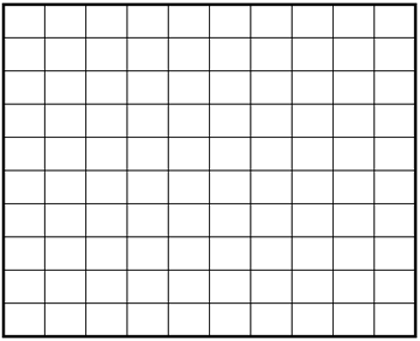2) Fill one of the squares with the number 1, then, based on the following two movement types, use consecutive numbers to fill in the rest of the grid:
Movement Type I: If the next number in the sequence is going to be placed vertically or horizontally, then it must be placed exactly three squares away from the previous number - there must be a two square gap between the numbers.

Movement Type II: If the next number in the sequence is going to be placed diagonally, then it must be placed exactly two squares away from the previous number - there must be a one square gap between the numbers.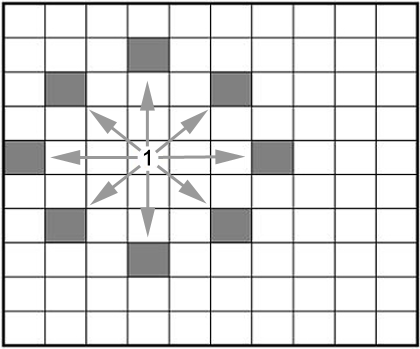3) Numbers can only be placed in empty squares - once a square has been used it can not be used again. All placements must occur within the grid.

4) Game is complete when the number 100 is reached - when all empty squares in the grid are filled. If you cannot continue placing numbers based on the two movement types above, and empty squares remain in the grid, then the puzzle is not complete and the game lost.

Two Incomplete Examples and a Solution
Example 1: The first example is one where I tried to get the worst possible result. I was able to end the game in 6 moves.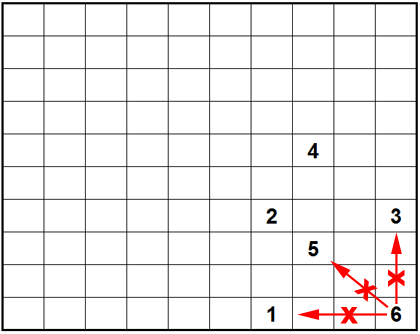Example 2: The second example is my best result so far. In this example I was able to reach 99 – one move short of finishing the game.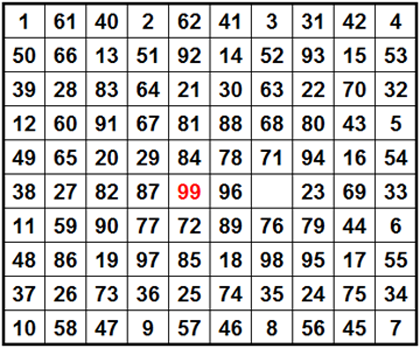A Solution: The final example is a solution completed by my student who introduced me to the game. He had been playing this game for approximately three years, and this was the only solution that he had been able to obtain during this period. This means that we know there will be at least four solutions to this game – the one presented below and its three mirrors. It would be interesting to find out how many solutions actually do exist - any mathematicians or programmers out there interested in tackling this problem?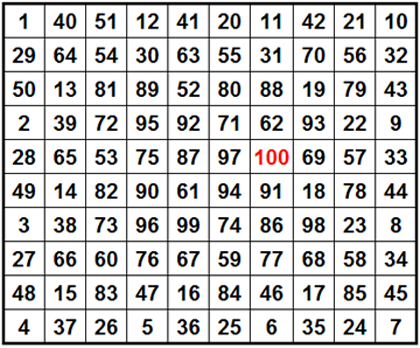Variations
Aside from creating a weighted scoring system or using symbols instead of numbers to fill in the squares, two variants could be: 1) give a starting point and an end point for the game – this will dramatically increase the difficulty of the puzzle; 2) create different types of grids but maintain the same placement rules – an example is presented below.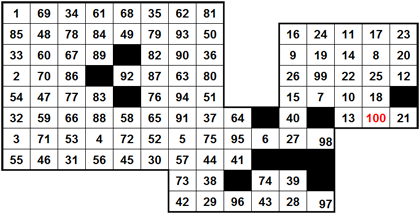For anyone who wants to try this out, below you will find a sheet with 12 grids (click to enlarge). Ideally, a simple program based on the parameters above loaded on a device with a touchscreen would be the best way to play this game. If you are into such things, I hope the game entertains you, and if you find anymore solutions, please send them my way. Thanks in advance.